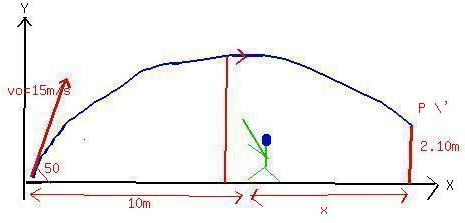Question# The lob in tennis is an effective tactic when your opponent is near the net. It consists of lofting the ball over his head, forcing him to move quickl

Other
ANSWEREDThe lob in tennis is an effective tactic when your opponent is near the net. It consists of lofting the ball over his head, forcing him to move quickly away from the net (see the drawing - I am sorry that I am not able to provide the drawing at this time as my scanner is broken). Suppose that your loft the ball with an initial speed of 15.0 m/s, at an angle of 50.0 (degrees) above the horizontal. At this instant your opponent is 10.0 m away from2021-02-26The components of the velocity are $$\displaystyle{v}_{{{o}{y}}}={v}_{{o}}{\sin{{50}}}={15}{\sin{{50}}}={11.5}\ \frac{{m}}{{s}}$$
$$\displaystyle{v}_{\otimes}={v}_{{o}}{\cos{{50}}}={9.64}\ \frac{{m}}{{s}}$$ The time taken by the ball to reach the point P ' is
$$\displaystyle{S}={v}_{{o}}{y}{t}-{\left({\frac{{{1}}}{{{2}}}}\right)}{>}^{{2}}$$
$$\displaystyle{0}{r}{2.10}={11.5}{t}-{\left({\frac{{{1}}}{{{2}}}}\right)}{\left({9.8}\right)}{t}^{{2}}$$
$$\displaystyle{4.9}{t}^{{2}}-{11.5}{t}+{2.1}={0}$$
This Quadractic equation in t, is solved for the values of t
$$\displaystyle{t}={2.15}\ {s}$$ and 0.2 s
as the opponent begins to move only after 0.3s later, we taket = 2.15s
Therefore, he catches the ball at time t'=(2.15-0.3)s
The total horizontal distance
$$\displaystyle{x}={v}_{\otimes}\cdot{t}$$
$$\displaystyle{\left({10}+{x}\right)}={v}_{\otimes}\cdot{2.15}{s}$$
$$\displaystyle={9.64}\cdot{2.15}'$$
The value of ' x ' is calculated.
Thus the required speed of the opponent $$\displaystyle{v}={\frac{{{x}}}{{{t}'}}}$$